# Logistic regression

## Objective

• Why the Linear regression model will not work for classification problems.
• How Logistic regression model is derived from a simple linear model.

## Introduction

While working with the machine learning models,  one question that generally comes into our mind for a given problem whether I should use the regression model or the classification model.

Regression and Classification both are supervised learning algorithms. In regression, the predicted values are of continuous nature and in classification predicted values are of a categorical type.

Note: If you are more interested in learning concepts in an Audio-Visual format, We have this entire article explained in the video below. If not, you may continue reading.

In simple terms, If you have a dataset with marks of a student in five subjects and you have to predict the marks in another subject it will be a regression problem. On the other hand, if I ask you to predict whether a student is pass or fail based on the marks it will be a classification problem.

Now let’s talk about Logistic regression. What do you think of what kind of algorithm is Logistic regression? A classification or a regression one.

##To our surprise, Logistic regression is actually a classification algorithm. Now you must be wondering if it is a classification algorithm why it is called regression.

Let’s consider a small example, here is a plot on the x-axis Age of the persons, and the y-axis shows they have a smartphone. It is a classification problem where given the age of a person and we have to predict if he posses a smartphone or not.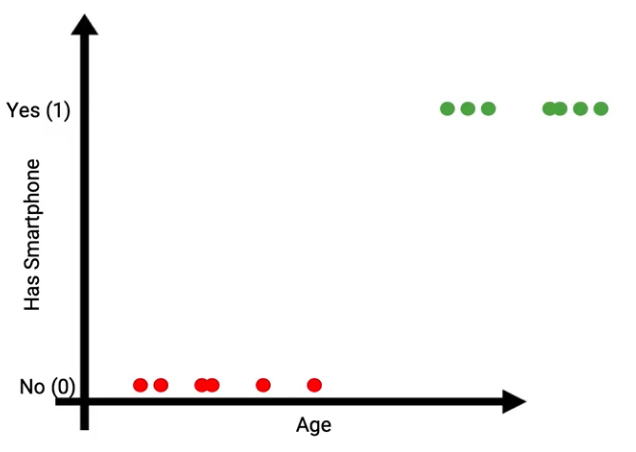In such a classification problem,  can we use linear regression?

## Issues with Linear regression

To solve the above prediction problem, let’s first use a Linear model. On the plot, we can draw a line that separates the data points into two groups. with a threshold Age value. All the data points below that threshold will be classified as 0 i.e those who do not have smartphones. Similarly, all the observations above the threshold will be classified as 1 which means these people have smartphones as shown in the image below.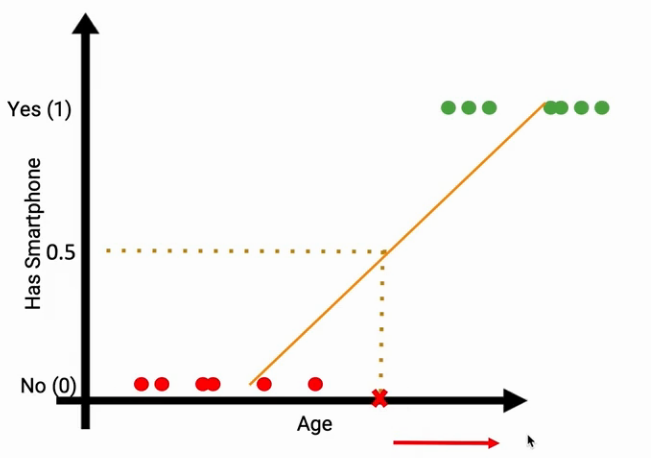Don’t you think it is successfully working? let me discuss some scenarios.

### Case 1

Suppose we got a new data point on the extreme right in the plot, suddenly you see the slope of the line changes. Now we have to inadvertently change the threshold of our model. Hence, this is the first issue we have with linear regression, our threshold of Age can not be changed in a predicting algorithm.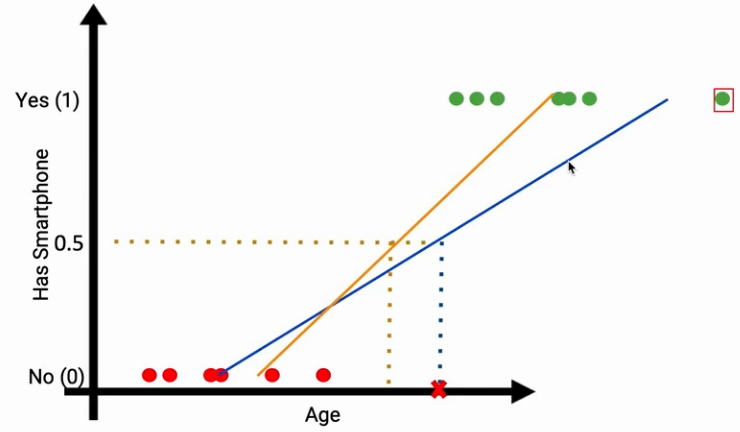### Case 2

The other issue with Linear regression is when you extend this line it will give you values above 1 and below 0. In our classification problem, we do not know what the values greater than one and below 0 represents. so it is not the natural extension of the linear model. Further, it makes the model interpretation at extremes a challenge.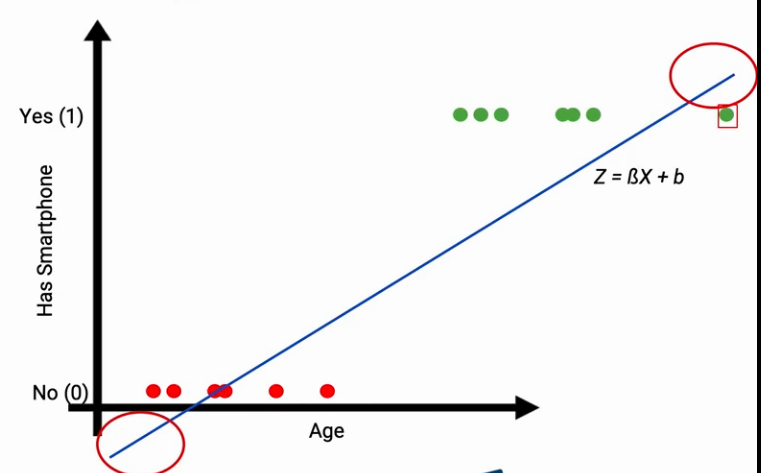## From Linear to logistic regression

Here the Logistic regression comes in. let’s try and build a new model known as Logistic regression. Suppose the equation of this linear line is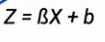Now we want a function Q( Z) that transforms the values between 0 and 1 as shown in the following image. This is the time when a sigmoid function or logit function comes in handy.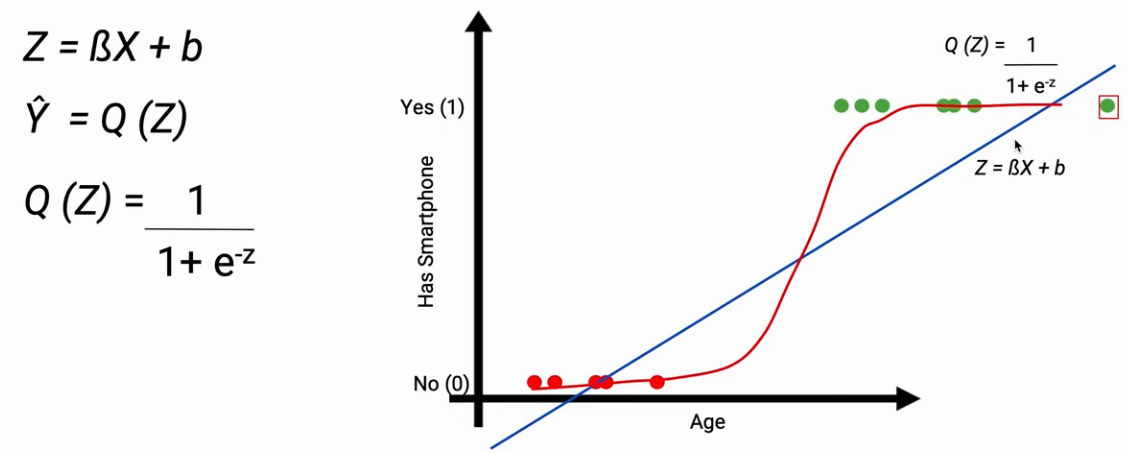This sigmoid function transforms the linear line into a curve. This will constraint the values between 0 and 1. Now it doesn’t matter how many new points I add to each extreme it will not affect my model.

The other important aspect is, for each observation model will give a continuous value between 0 and 1. This continuous value is the prediction probability of that data point. If the prediction probability is near 1 then the data point will be classified as 1 else 0.

## The cost function for Logistic regression

For linear regression, the cost function is mostly we use Mean squared error represented as the difference y_predicted and y_actual iterated overall data points, and then you do a square and take the average. It is a convex function as shown below. This cost function can be optimized easily using gradient descent.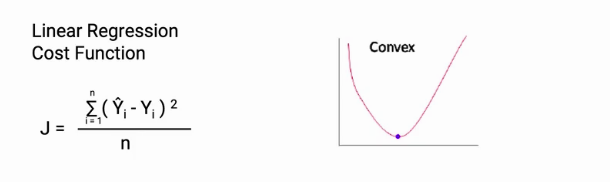Whereas, If we use the same cost function for the Logistic regression is a non-linear function, it will have a non-convex plot. It will create unnecessary complications if use gradient descent for model optimization.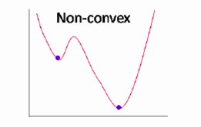Hence, we need a different cost function for our new model. Here comes the log loss in the picture.  As you can see, we have replaced the probability in the log loss equation with y_hat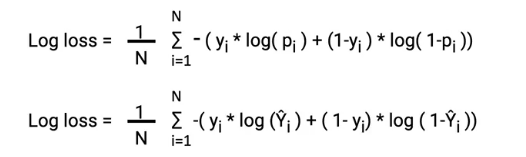In the first case when the class is 1 and the probability is close to 1, the left side of the equation becomes active and the right part vanishes. You will notice in the plot below as the predicted probability moves towards 0 the cost increases sharply.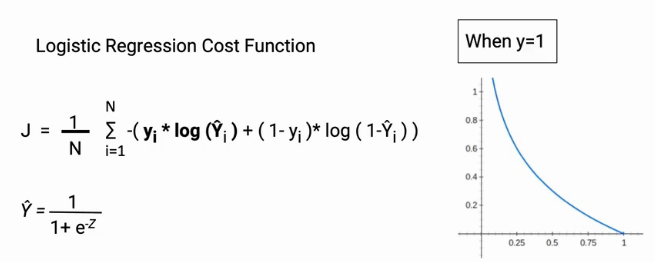Similarly, when the actual class is 0 and the predicted probability is 0, the right side becomes active and the left side vanishes. Increasing the cost of the wrong predictions. Later, these two parts will be added.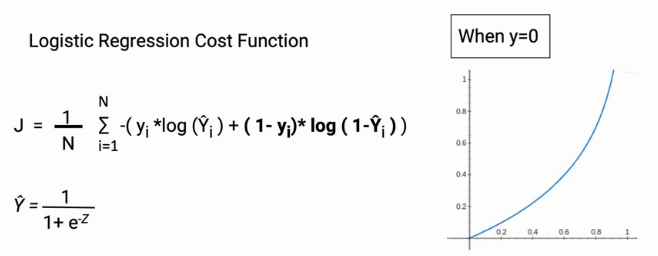To understand log loss in detail, I will suggest you go through this article Binary Cross Entropy/Log Loss for Binary Classification

## Optimize the model

Once we have our model and the appropriate cost function handy, we can use “The Gradient Descent Algorithm” to optimize our model parameters. As we do in the case of linear regression.

Although gradient descent is a separate topic still I will quickly explain it as shown in the following image. We have the cost function J and the parameters B and b. Now we can differentiate the cost function J with parameters B and b. Once we have our values of GB and Gb, we can update our parameters.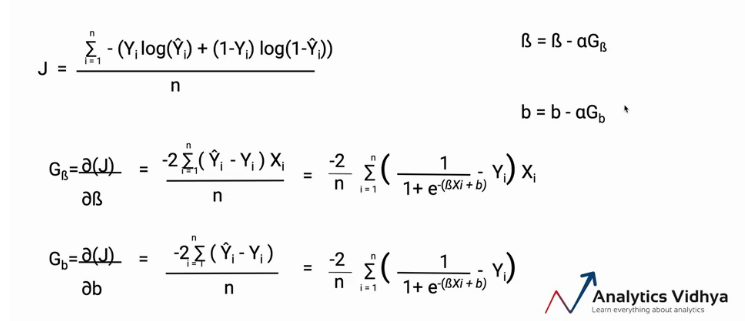The whole process will go iteratively until we get our best parameters.

To understand how gradient descent algorithms work please go through the following article-

Understanding the Gradient Descent Algorithm

## Endnote

To summarise, in this article we learn why linear regression doesn’t work in the case of classification problems and the issues. Also, how the logistic regression is different from linear regression and it resolves the challenges of the linear models.

If you are looking to kick start your Data Science Journey and want every topic under one roof, your search stops here. Check out Analytics Vidhya’s Certified AI & ML BlackBelt Plus Program

Let us know if you have any queries in the comments below.